# Positive Displacement Pump Element

## Positive Displacement Pump General Description & Quick Guide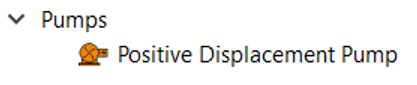The Positive Displacement pump element routine simulates only for Incompressible Liquids. In Principle the Positive displacement pump behaves like a Fixed Flow element where the flow gets calculated from volumetric displacement provided by manufacturer for the specific pump. The PD pump model is based on a non-dimensional loss coefficient model developed by Wilson. (Ref: Wilson, W. “Performance criteria for positive displacement pumps and fluid motors”, ASME Semi-annual Meeting, paper No. 48-SA-14, 1948.

## Positive Displacement Pump Element Inputs

Table of the inputs for the Positive Displacement Element.

Element Specific Positive Displacement Pump Element Input Variables
Index Field Description
1 Pump Type (SUBTYPE)

Positive displacement pump subtypes. (No Meaning)

Default =1

2 Speed (SPEED) Pump shaft rotation speed in revolutions per minute
3 Volumetric Displacement (VOL_DISP) Maximum Volumetric Displacement per cycle
4 Pump Leakage Coefficient (LEAK_COEFF) The leakage coefficient characterizes the leakage flow which occurs within the pump. (Unitless)
5 Coulomb Friction Coefficient (COULOMB_COEFF) Coulomb Friction Coefficient is the friction between the moving part (gear, vane, or piston) on the stationary part (casing or cylinder). (Unitless)
6 Viscous Friction Coefficient (VISCOUS_COEFF) Viscous Friction Coefficient is the friction between the moving fluid and the casing or cylinder wall (Unitless)
7 Flow Efficiency Option (LEAK_OPT)

There are two ways to specify flow efficiency:

1: Fixed Leakage Coefficient

2: Leakage Coefficient vs. Speed

3: Leakage Coefficient vs. Delta.P vs. Speed

11: Fixed Volumetric Efficiency

12: Volumetric Efficiency vs. Speed

13: Volumetric Efficiency vs. Delta.P vs. Speed

8 Volumetric Efficiency Fixed Volumetric Efficiency
10 Exit Area (EXIT_AREA) Area at the pump’s exit. This input helps to account for dynamic pressure exiting the pump.
12 Element Inlet Orientation: Tangential Angle (THETA)

Angle between the element centerline at the entrance of the element and the reference direction.

If the element is rotating or directly connected to one or more rotating elements, the reference direction is defined as parallel to the engine centerline and the angle is the projected angle in the tangential direction. Otherwise, the reference direction is arbitrary but assumed to be the same as the reference direction for all other elements attached to the upstream chamber.

THETA for an element downstream of a plenum chamber has no impact on the solution except to set the default value of THETA_EX.

13 Element Inlet Orientation: Radial Angle (PHI)

Angle between the element centerline at the entrance of the element and the THETA direction. (spherical coordinate system)

PHI for an element downstream of a plenum chamber has no impact on the solution except to set the default value of PHI_EX.

14 Element Exit Orientation: Tangential Angle (THETA_EX)

Angle between the orifice exit centerline and the reference direction.

THETA_EX is an optional variable to be used if the orientation of the element exit differs from that of the element inlet.

The default value (THETA_EX = -999) will result in the assumption that THETA_EX = THETA.

Other values will be interpreted in the manner presented in the description of THETA.

15 Element Exit Orientation: Radial Angle (PHI_EX)

Angle between the orifice exit centerline and the THETA_EX direction.

PHI_EX is an optional variable to be used if the orientation of the element exit differs from that of the element inlet.

The default (PHI_EX = -999) will result in the assumption that PHI_EX = PHI.

Other values will be interpreted in the manner presented in the description of PHI.

16 Fluid Compressibility Mode (FLUID_MODE)

The user can choose which solution algorithm to use.

By Default, 2: Incompressible Liquid

17 Mechanical Efficiency Option (MECH_EFF_OPT)

Ways to specify Mechanical efficiency:

0: Friction Coefficients

11: Fixed Mechanical Efficiency

12: Mechanical Efficiency vs. Speed

13: Mechanical Efficiency vs. Delta.P vs. Speed

18 Mechanical Efficiency (MECH_EFF) Fixed Mechanical Efficiency
19 TBL1_RPM/ TBL2_RPM/ TBL3_SPEED/ TBL4_SPEED

Independent variable curve for shaft speed

Should be in ascending order

20 TBL1_LEAK_COEFF/ TBL2_LEAK_COEFF

Dependent variable curve for leakage coefficient

Unitless

21 TBL2_DELTAP/ TBL4_DELTAP Independent variable curve Delta Pressure Rise across Pump
22 TBL3_VOL_EFF /TBL4_VOL_EFF Dependent variable curve Volumetric Efficiency Table

## Positive Displacement Theory Manual

Mass Flow rate Calculation

The Flow rate for a give pump is given by

If Leakage Coefficient is provided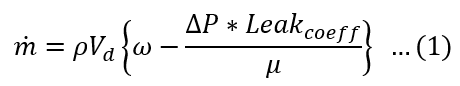If Volumetric Efficiency is provided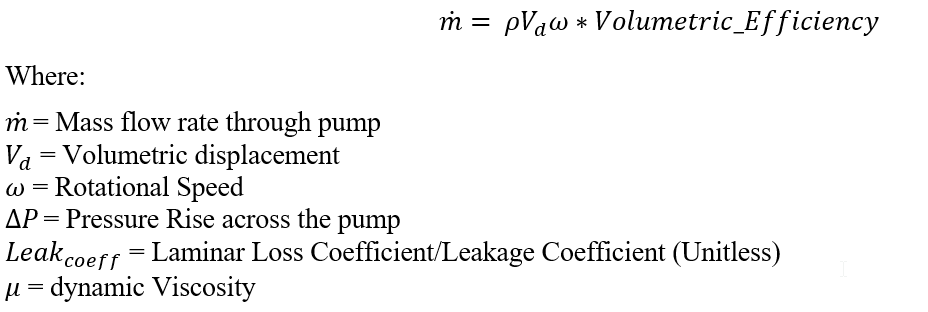Addition to this the efficiencies & torque across the pump is also calculated.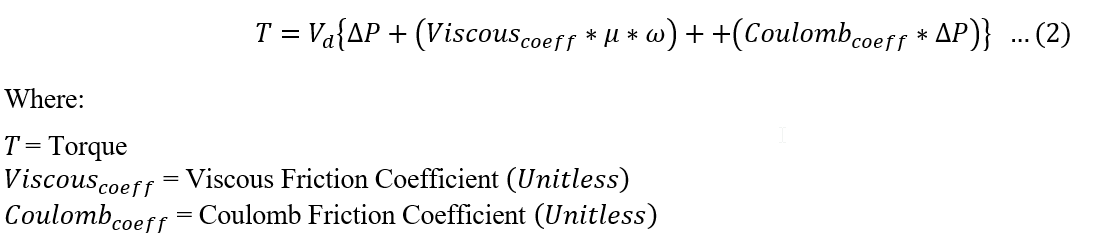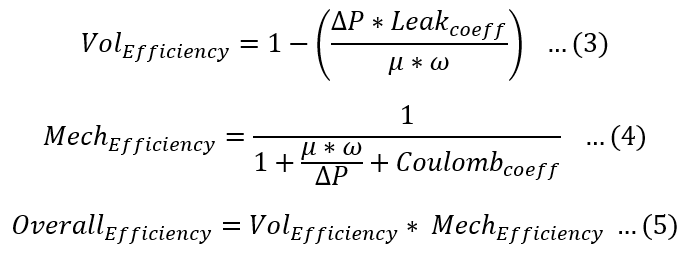For Additional Momentum loss, Portion of Upstream Dynamic Head loss, refer Solver General theory section.

## Positive Displacement Element Outputs

The following listing provides details about conventional orifice output variables.

Name Description Units
DQ_IN

Portion of Ustrm Chamb. Dyn. Head Lost

(Usually an echo of the user input unless modified inside Flow Simulator.)

Flag

Axial (K_EXIT_Z)

Tangential (K_EXIT_U)

Exit K Loss

(Usually an echo of the user input unless modified inside Flow Simulator.)

Unitless
ELEMENT_THETA

Tangential Angle

(Usually an echo of the user input but converted to radians.)

ELEMENT_PHI

(Usually an echo of the user input but converted to radians.)

REL_INLET_ANGLE It is a relative inlet angle calculated based on upstream chamber velocity Deg
IDEAL_FLOW (Total flow – Leakage flow) Lbm/s, Kg/s
SPPED

Pump Speed

(Usually an echo of the user input unless modified inside Flow Simulator.)

RPM
LEAKAGE_FLOW Leakage Flow Lbm/s, Kg/s
EXIT_AREA

Exit area used for calculating exit conditions of the orifice element. This output is only printed when an exit area is used (EXIT_AREA>0). A default value of 0 has no effect on exit conditions.

(Output is an echo of the user input.)

inch2, m2
VOL_EFFIC Volumetric Efficiency (Unitless)
LEAKAGE_COEFF Leakage Coefficient (Unitless)
MECH_EFFIC Mechanical Efficiency (Unitless)
COULOMB_COEFF Coulomb Friction Coefficient. (Unitless)
VISCOUS_COEFF Viscous Friction Coefficient (Unitless)
HYDRAULIC_POWER Hydraulic Power HP
SHAFT_HPOWER Shaft Power HP
OVERALL_EFFIC Overall Efficiency (Unitless)
TORQUE Torque Ft-lbm, N-m
PTS Driving pressure relative to the rotational reference frame (i.e. rotor) at the restriction inlet. psi, mPa
PTEX Total pressure relative to the rotational reference frame (i.e. rotor) at the restriction exit including supersonic effects. psi, mPa
PSEX

Static pressure relative to the rotational reference frame (i.e. rotor) at the restriction exit.

Limited by critical pressure ratio for supersonic flows.

psi, mPa
PSEB Effective sink (static) pressure downstream of the restriction. psi, mPa
TTS Total temperature of fluid relative to the rotational reference frame (i.e. rotor) at the restriction inlet. deg F, K